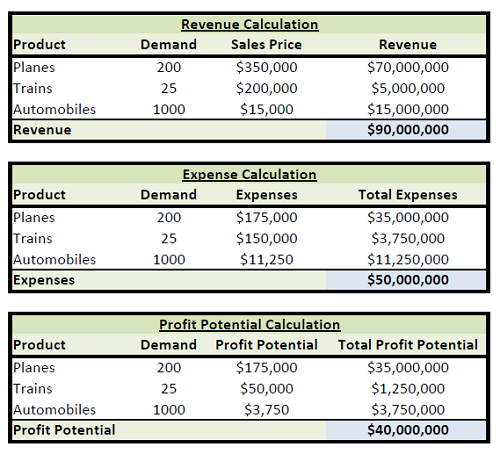# Profit Potential: Definition & Overview

Michael is a financial planner and has a master's degree in financial services.

A product with profit potential will generate revenue, creating income. Explore the definition of profit potential with an overview of the concept and how to calculate potential for multiple products. Updated: 02/03/2022

## Definition

Profit potential is the potential for a product to generate revenue which, after expenses, leads to net income. It's important to highlight the importance of the word 'potential.' Think of this as a projection, not a guarantee.

Let's start the lesson with a simple example which we can expand upon. Let's say a business owner decides to place a sale on widgets. Prior to beginning the sale, the inventory count is 100 units. The sale price is \$5 per widget, and expenses amount to \$3 per unit.

Profit potential is then:

100 units in inventory x (sale price of \$5 per unit - expenses of \$3 per unit) = \$200 profit potential

Put another way:

I x (P-E) = PP, where:

I = inventory or potential demand, in units

P = sale price per unit

E = expenses per unit

PP = profit potential

It should also be noted that inventory (or potential demand) multiplied by sale price per unit equates to expected revenue. Expected revenue less expenses, therefore, equals profit potential.

## Calculating Profit Potential for Multiple Products

Let's dig a bit deeper into the concept but using an example with the fictitious transportation company, ABC Industries. ABC is the maker of planes, trains, and automobiles. Unfortunately for the consumer, ABC Industries only produces a standard version of each product. ABC Industries accounts for the expenses of each product separately.

Planes:

Sale price of \$350,000 per plane

Projected demand of 100 units this year

Expenses are projected to be 50% of the sales price

Trains:

Sale price of \$200,000 per box car

Projected demand of 50 units this year

Expenses are projected to be \$150,000 per unit

Automobiles:

Sale price of \$15,000 per car

Projected demand of 1,000 units this year

Expenses are projected to be 75% of the sales price

In the next year, ABC Industries expects sales of planes to double, while sales of trains to decline by 50%. Automobile sales are expected to remain constant. ABC does not intend to alter the price of the products, nor do they expect expenses per unit to change. Let's calculate next year's profit potential for the owners of ABC Industries.ABC Industries can review the gross margin percentage on each of their products. Gross margin percentage is the percentage of profit to revenue. Gross margin can be calculated as:

Planes: \$35,000,000 / \$70,000,000 = 50% gross margin percentage

Trains: \$1,250,000 / \$5,000,000 = 25% gross margin percentage

Automobiles: \$3,750,000 / \$15,000,000 = 25% gross margin percentage

Overall: \$40,000,000 / \$90,000,000 = 44.44% gross margin percentage

To unlock this lesson you must be a Study.com Member.

### Register to view this lesson

Are you a student or a teacher?

Back

### Resources created by teachers for teachers

Over 30,000 video lessons & teaching resources‐all in one place.Video lessonsQuizzes & WorksheetsClassroom IntegrationLesson Plans

I would definitely recommend Study.com to my colleagues. It’s like a teacher waved a magic wand and did the work for me. I feel like it’s a lifeline.

Jennifer B.
TeacherCreate an account to start this course today
Used by over 30 million students worldwide